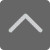## 爱智康资讯站

### 课程咨询: 4000-121-121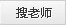# 初中物理公式整合 期末复习再也不怕

2019-01-07 09:57:24 　来源：网络整理

这个学期快要余额不足，期末的脚步越来越近。

复习，你还在埋头做题吗?

物理，作为不少同学学习的难点区域，

除了对自身错题进行针对性的练习，

自身的知识漏洞也要补一补。

公式定理需要记忆和背诵

ps.因为格式问题，有些上标和下标未能正常显示，请大家注意。

力学部分

一、速度公式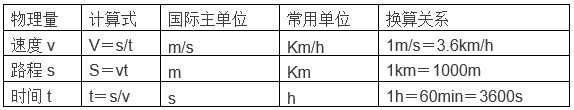火车过桥(洞)时通过的路程s=L桥+L车

声音在空气中的传播速度为340m/s

光在空气中的传播速度为3×108m/s

二、密度公式

(ρ水=1.0×103 kg/m3)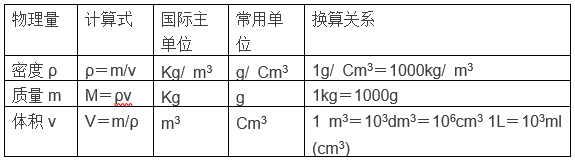冰与水之间状态发生变化时m水=m冰 ρ水>ρ冰 v水

同一个容器装满不同的液体时，不同液体的体积相等，密度大的质量大

空心球空心部分体积V空=V总-V实

三、重力公式

G=mg (通常g取10N/kg，题目未交待时g取9.8N/kg)

同一物体 G月=1/6G地

m月=m地

四、杠杆平衡条件公式

F1l1=F2l2

F1 /F2=l2/l1

五、动滑轮公式

不计绳重和摩擦时F=1/2(G动+G物)s=2h

六、滑轮组公式

不计绳重和摩擦时F=1/n(G动+G物)s=nh

七、压强公式(普适)

P=F/S

固体平放时F=G=mg

S的国际主单位是m2 1m2=102dm2=104cm2=106mm2

八、液体压强公式

P=ρgh

液体压力公式F=PS=ρghS

规则物体(正方体、长方体、圆柱体)公式通用

九、浮力公式

(1)F浮=F’-F (压力差法)

(2)F浮=G-F (视重法)

(3)F浮=G (漂浮、悬浮法)

(4)阿基米德原理：F浮=G排=ρ液gV排 (排水法)

十、功的公式

W=FS

把物体举高时W=GhW=Pt

十一、功率公式

P=W/t

P=W/t=Fs/t=Fv(v=P/F)

十二、有用功公式

举高W有=Gh

水平W有=Fs

W有=W总-W额

十三、总功公式

W总=FS (S=nh)

W总=W有/η

W总= W有+W额

W总=P总t

十四、机械效率公式

η=W有/W总 η=P有/ P总

(在滑轮组中η=G/Fn)

(1)η=G/ nF(竖直方向)

(2)η=G/(G+G动) (竖直方向不计摩擦)

(3)η=f / nF (水平方向)

十五、热学公式

C水=4.2×103J/(Kg·℃)

1.吸热：Q吸=Cm(t-t0)=CmΔt

2.放热：Q放=Cm(t0-t)=CmΔt

3.热值：q=Q/m

4.炉子和热机的效率： η=Q有效利用/Q燃料

5.热平衡方程：Q放=Q吸

6.热力学温度：T=t+273K

7.燃料燃烧放热公式Q吸=mq或Q吸=Vq(适用于天然气等)

电学部分

1.电流强度：I=Q电量/t

2.电阻：R=ρL/S

3.欧姆定律：I=U/R

4.焦耳定律：

(1)Q=I2Rt普适公式)

(2)Q=UIt=Pt=UQ电量=U2t/R (纯电阻公式)

5.串联电路：

(1)I=I1=I2

(2)U=U1+U2

(3)R=R1+R2

(5)W=UIt=Pt=UQ (普适公式)

(6)W=I2Rt=U2t/R (纯电阻公式)

(7)U1/U2=R1/R2 (分压公式)

(8)P1/P2=R1/R2

6.并联电路：

(1)I=I1+I2

(2)U=U1=U2

(3)1/R=1/R1+1/R2 [ R=R1R2/(R1+R2)]

(4)I1/I2=R2/R1(分流公式)

(5)P1/P2=R2/R1

7.定值电阻：

(1)I1/I2=U1/U2

(2)P1/P2=I12/I22

(3)P1/P2=U12/U22

8.电功

(1)W=UIt=Pt=UQ (普适公式)

(2)W=I2Rt=U2t/R (纯电阻公式)

9.电功率：

(1)P=W/t=UI (普适公式)

(2)P=I2R=U2/R (纯电阻公式)

常用物理量

1光速：C=3×108m/s (真空中)

2.声速：V=340m/s (15℃)

3.人耳区分回声：≥0.1s

4.重力加速度g=9.8N/kg≈10N/kg

5.标准大气压值：760毫米水银柱高=1.01×105Pa

6.水的密度：ρ=1.0×103kg/m3

7.水的凝固点：0℃

8.水的沸点：100℃

9.水的比热容：C=4.2×103J/(kg•℃)

10.元电荷：e=1.6×10-19C

11.一节干电池电压：1.5V

12.一节铅蓄电池电压：2V

13.对于人体的安全电压：≤36V(不高于36V)

14.动力电路的电压：380V

15.家庭电路电压：220V

16.单位换算：

(1)1m/s=3.6km/h

(2)1g/cm3 =103kg/m3

(3)1kw•h=3.6×106J

以上就是为大家整理的初中物理公式整合 期末复习再也不怕。同学们还有其他学习上的问题，可拨打爱智康课程免费咨询热线电话：4000-121-121 .那里有专业的老师为大家解答。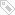标签：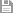保存　｜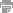打印　｜关闭